# KSEEB SSLC Class 10 Maths Solutions Chapter 5 Areas Related to Circles Ex 5.1

In this chapter, we provide KSEEB SSLC Class 10 Maths Solutions Chapter 5 Areas Related to Circles Ex 5.1 for English medium students, Which will very helpful for every student in their exams. Students can download the latest KSEEB SSLC Class 10 Maths Solutions Chapter 5 Areas Related to Circles Ex 5.1 pdf, free KSEEB SSLC Class 10 Maths Solutions Chapter 5 Areas Related to Circles Ex 5.1 book pdf download. Now you will get step by step solution to each question.

### Karnataka State Syllabus Class 10 Maths SolutionsChapter 5 Areas Related to Circles Ex 5.1

Question 1.
The radii of two circles are 19 cm and 9 cm respectively. Find the radius of the circle which has circumference equal to the sum of the circumferences of the two circles.
Solution:
Radius of 1st Circle, r1 = 19 cm.
Circumference of 1st circle, C1 = 2πr1
=2×227×19
Radius of 2nd circle, r2 = 9 cm.
Circumference of 2nd circle, C2 = 2πr2
=2×227×9
Sum of the circumferences of the two circles
=2×227×19+2×227×9
C3=2×227(19+9)
=2×227×28
= 2 × 22 × 4
∴ C3 = 176 cm.
Circumference of 3rd circle, C3 = 176 cm. then, r= ?
2πr3 = C3
2×227×r3=176
r3=176×722×12
∴ r3 = 28 cm

Question 2.
The radii of two circles are 8 cm and 6 cm respectively. Find the radius of the circle having area equal to the sum of the areas of the two circles.
Solution:
Radius of 1st Circle, r1 = 8 cm.
Area of 1st Circle, A1 = π r12
=227×(8)2
A1=227×64
Radius of 2nd Circle, r2 = 6 cm.
Area of 2nd Circle, A2 = π r22
=227×(6)2
A2=227×36
Total area of two circles =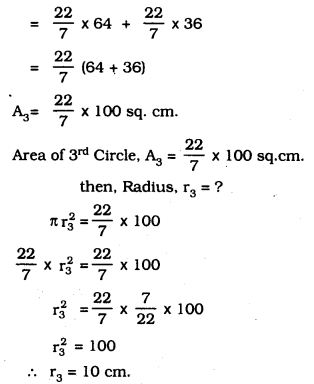Question 3.
The figure given below depicts an archery target marked with its five scoring regions from the centre outwards as Gold, Red, Blue, Black, and White. The diameter of the region representing the Gold score is 21 cm. and each of the other bands is 10.5 cm. wide. Find the area of each of the five scoring regions.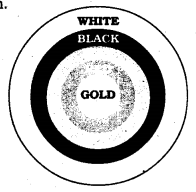Solution:
i) The diameter of the region representing Gold Score :
21
d = 21 cm. ∴ Radius, r=212cm
∴ Area of the region representing Gold score
= πr2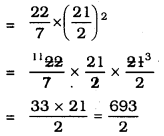A = 346.5 sq. cm.

ii) Radius of the region representing Gold score and Red score:
r = 10.5 + 10.5 = 21 cm.
∴ Area of the region representing Red score: Area of the region representing Gold and Red Score — Area of the region representing Gold score.
= πr2 – πr2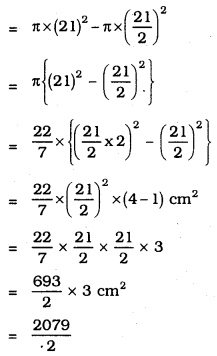∴ A = 1039.5 sq.cm.

iii) Total radii of the region representing Gold, Red and Blue region :
r =10.5 + 10.5 + 10.5 = 31.5 cm
=632cm
∴ Area of the region representing Blue region:
[ Area of the region representing Gold, Red and Blue regions ] — Area of the region representing Gold and Red region
∴ A = πr2 – πr2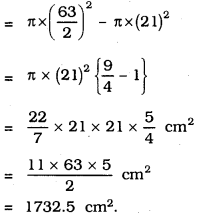iv) Similarly, to find Area of the region representing Black region :
∴ A = πr2 – πr2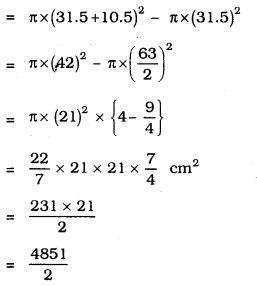∴ A = 2425.5 sq. cm.

v) Area of the region representing white:
∴ A = πr2 – πr2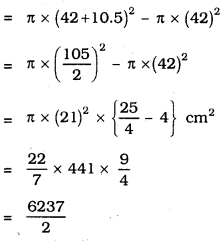∴ A = 3118.5 sq. cm

Question 4.
The wheels of a car are of diameter 80 cm each. How many complete revolutions does each wheel make in 10 minutes when the car is travelling at a speed of 66 km per hour?
Solution:
Diameter of the Wheel of the car = 80 cm.
Radius of the wheel = 40 cm.
Circumference of the Wheel = 2πr
=2×227×40
=44×407
= 251.4 cm
Speed of the car = 66 km/hr.
=66×10000060cm./min
= 110000 cm/min.
Distance travelled by car in 10 minutes
= 110000 × 10
= 1100000 cm.
Let the number of revolutions of the wheel be ’n’, then
n × 251.4 = 1100000
∴ n=1100000251.4
n = 4375
∴ Car can complete 4,375 revolutions in 10 minutes.

Question 5.
Tick the correct answer in the following and justify your choice: If the perimeter and the area of a circle are numerically equal, then the radius of the circle is
A) 2 units
B) n units
C) 4 units
D) 7 units
Solution:
A) 2 units
Circumference of a circle = Area of the circle.
2πr = πr2
2 × π × r = π × r × r
∴ 2 = r
∴ r = 2 units.

All Chapter KSEEB Solutions For Class 10 Maths

—————————————————————————–

All Subject KSEEB Solutions For Class 10

*************************************************

I think you got complete solutions for this chapter. If You have any queries regarding this chapter, please comment on the below section our subject teacher will answer you. We tried our best to give complete solutions so you got good marks in your exam.

If these solutions have helped you, you can also share kseebsolutionsfor.com to your friends.

Best of Luck!!